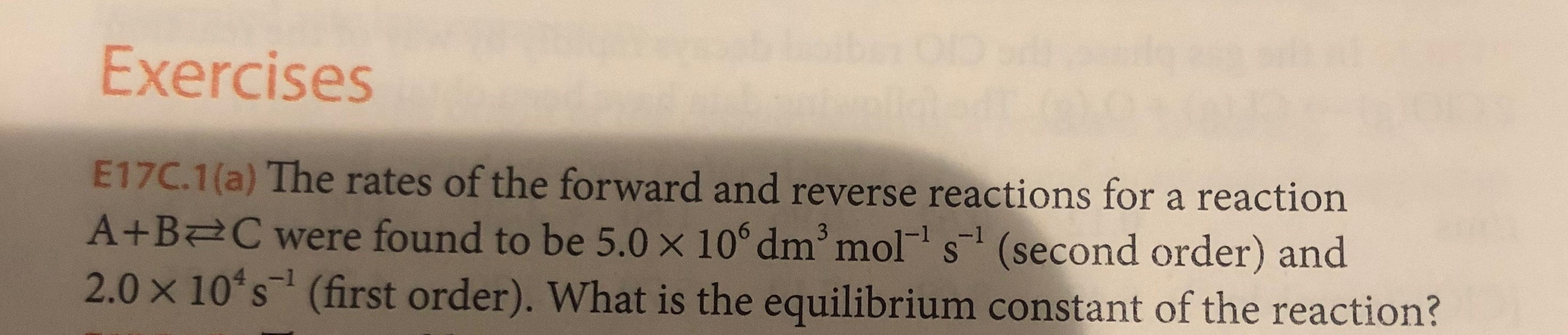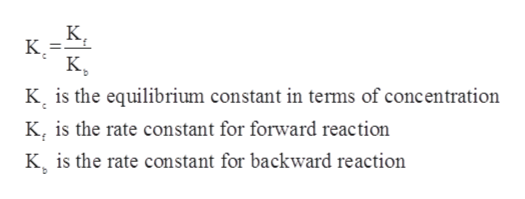# ExercisesE17C.1(a) The rates of the forward and reverse reactions for a reactionA+B C were found to be 5.0 x 10° dm mol s (second order) and2.0 x 10 s (first order). What is the equilibrium constant of the reaction?3

Question
1 viewshelp_outlineImage TranscriptioncloseExercises E17C.1(a) The rates of the forward and reverse reactions for a reaction A+B C were found to be 5.0 x 10° dm mol s (second order) and 2.0 x 10 s (first order). What is the equilibrium constant of the reaction? 3 fullscreen
check_circle

Step 1

The equilibrium constant for a given chemical reaction can be defined as the reaction quotient of that reaction at equilibrium and it can be sued to find out the concentrations of each of the components of a reversible reaction at equilibrium. The mathematical relation between the equilibrium constant and the rate of forward and backward reaction is as follows:help_outlineImage TranscriptioncloseК, K =-** К, K is the equilibrium constant in terms of concentration K, is the rate constant for forward reaction K is the rate constant for backward reaction fullscreen
Step 2

In the given problem, the rate of the forward reaction is given to be 5.0×106 dm3 mol-1s-1.

The rate of the backward reaction is given to be 2.0&t...

### Want to see the full answer?

See Solution

#### Want to see this answer and more?

Solutions are written by subject experts who are available 24/7. Questions are typically answered within 1 hour.*

See Solution
*Response times may vary by subject and question.
Tagged in

### Physical Chemistry